www.batmath.it

### Saying maths 3 - Logic and Sets, Functions

#### Logic and setsthere exists! there exists only onep non p / not p | such that (in the definition of sets by listing)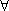for all / for any p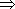q p implies q / if p then q p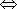q p if and only if q / p is equivalent to q / p and q are equivalent xA x is an element of A / x belongs to A xA x is not an element of A / x does not belong to A U universal setempty set A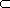B A is (properly) contained in B /  A is a (proper) subset of B A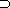B A (properly) contains B / B is a (proper) subset of A A ∩ B A intersection B / A meet B / A cap B A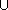B A union B / A join B / A cup B A \ B A minus B / the difference between A and B Ac  or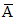the complement of A A × B A cross B / the Cartesian product of A and B P(A)= {0,1}A the power set of A / the set of all subsets of a set A (a,b) the ordered pair a b

#### Functions and analysis

 ex e to the x / the exponential function lnx natural logarithm of x / natural log of x / log base e of x / ln of x ax a to the x / the exponential function base a logax log base a of x / log x base a sinx sine x / sine of x cosx cosine x / cosine of x tanx tangent x / tangent of x arcsinx arcsine x / arcsine of x / inverse sine of x arccosx arccosine x / arccosine of x / inverse cosine of x arctanx arctangent x / arctangent of x / inverse tangent of x f : S → T function f from S to T S is the domain, T the range (rarely the codomain) f(A)  ;  f(X) the image of A  ;  the image of the domain or simply the image (observe that, as in Italian, there is no general agreement about these terms: range is often used in the place of  image - we do not agree with this) f-1(B) the inverse image of B / the pre-image of B f : x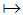y f maps x to y xy x maps to y / x is sent (or mapped) to y f(x) f x / f of x / the function f of x f-1(x) f inverse  `-pause-` of x f ' f prime / f dash / the derivative of f / the first derivative of f f '(x) f prime (of) x / f dash (of) x / the derivative of f with respect to x / the first derivative of f with respect to x f '' f double-prime / f double-dash / the second derivative of f f ''(x) f double-prime (of) x / f double-dash (of) x / the second derivative of f with respect to x f '''  ; f '''(x) the same as f ' or f '(x) with triple-prime or triple-dash in the place of prime or dash f(n) f n / the nth derivative of f f(n)(x) f n (of) x / the nth derivative of f with respect to x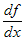d f d x  / see f '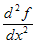d squared f `-pause-` (over) d x squared / see f'' or f''(x)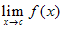limit as x tends to c of f x / limit as x approaches c of f x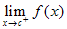... tends to c from above... / ... approaches c from above ...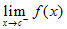... tends to c from below... / ... approaches c from below ... ∞  ;  +∞  ;  -∞ infinity (while infinite is an adjective)  ; plus infinity  ;  minus infinity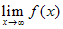limit as x tends to infinity of f x / limit as x goes to infinity of f x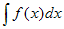the indefinite integral of f x d x / the antiderivative of f x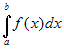the definite integral of f x d x from a to b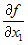the (first) partial derivative of f with respect to x1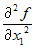the second partial derivative of f with respect to x1 Terms about functions surjection / surjective map / onto map injection / injective map bijection / bijective map / one-to-one map composition map piecewise defined map
first published on february 08 2003 - last updated on september 01 2003